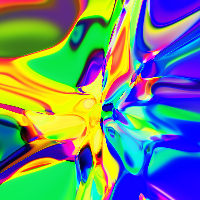# For: Batch Normalization: Accelerating Deep Network Training by Reducing Internal Covariate Shift

560

1. 摘要
2. 背景
3. Batch Normalization
4. 实验
5. 其它
6. 引用

## 摘要

Internal covariate shift/covariate shift 这里中文随手翻译的，看得懂意思就行。

## Batch Normalization

$$\hat{x}^{(k)} = \frac{x^{(k)} - E[x^{(k)}]}{\sqrt{Var[x^{(k)}]}}, k = 1, 2, ..., d$$$$y^{(k)} = \gamma^{(k)}\hat{x}^{(k)} + \beta^{(k)}$$

\begin{align} \gamma^{(k)} &= \sqrt{Var[x^{(k)}]}\\ \beta^{(k)} &= E[x^{(k)}] \end{align}

### BN 算法

\begin{align} \mu_{\mathcal{B}} & \leftarrow\frac{1}{m}\sum_{i=1}^m x_i\\ \sigma_{\mathcal{B}}^2 & \leftarrow\frac{1}{m}\sum_{i=1}^m(x_i - \mu_{\mathcal{B}})^2\\ \hat{x}_i & \leftarrow \frac{x_i - \mu_{\mathcal{B}}}{\sqrt{\sigma_{\mathcal{B}}^2 + \epsilon}}\\ y_i & \leftarrow \gamma \hat{x}_i + \beta \equiv BN_{\gamma, \beta}(x_i) \end{align}

BN的每一步都是可导的，因此很容易集成到深度学习网络中去。应用BN之后，假设原先每一层的输入为$x$，那么之后就变成了$BN(x)$。

$$\hat{x} = \frac{x - E[x]}{\sqrt{Var[x] + \epsilon}}$$

1. 设计一个神经网络$N_{\Theta}$, 其中$\Theta$为网络的参数
2. 在需要的地方插入BN层，这样得到训练用网络$N_{BN}$
3. 正常训练$N_{BN}$
4. 训练完成后，对于每一个 batch $\mathcal{B_i}, i = 1, 2, ..., m$ (假设我们有 m 个 batch), 计算得到 BN的输入$x$的均值集合$\{\mu_{\mathcal{B_i}}\}$以及方差集合$\{ \sigma_{\mathcal{B_i}}^2 \}$
5. 计算$E[x]$和$Var[x]$:
\begin{align} E[x] & = \frac{1}{m} \sum_{i=1}^{m}\mu_{\mathcal{B_i}}\\ Var[x]& = \frac{m}{m-1}(\frac{1}{m}\sum_{i=1}^m \sigma_{\mathcal{B}_i}^2) \end{align}

### 在CNN中使用

\begin{align} E[x] & = \frac{1}{m^{\prime}} \sum_{i=1}^{m^{\prime}}\mu_{\mathcal{B_i}}\\ & = \frac{1}{m\times p \times q} \sum_{i=1}^{m\times p \times q}\mu_{\mathcal{B_i}} \end{align}

$Var(x)$求法也一样，是对$m^{\prime}$个数据求的。

### 使用更大的学习率

$$BN((aW)u) = BN(Wu)$$

$$\frac{\partial BN((aW)u)}{\partial u} = \frac{\partial BN(Wu)}{\partial u}$$

## 实验### 使用参考

1. 提高了学习率
2. 去除dropout
3. 对于训练数据进行更加彻底的打乱 (为了得到更具代表性的batch数据)
4. 减少 $L_2$正则系数
5. 提高学习率衰减系数
6. 移除*Local Response Normalization*
7. 减少在图片上的光学上的调整，让网络多看到更多”真实“的图片

## 引用

 Ioffe, Sergey, and Christian Szegedy. "Batch normalization: Accelerating deep network training by reducing internal covariate shift." International conference on machine learning. PMLR, 2015.

 Batch Normalization before or after ReLU? https://www.reddit.com/r/MachineLearning/comments/67gonq/d_batch_normalization_before_or_after_relu/

 Santurkar, Shibani, et al. "How does batch normalization help optimization?." arXiv preprint arXiv:1805.11604 (2018).

Article Tags
[本]通信工程@河海大学 & [硕]CS@清华大学

1
560
0

More RecommendationsNov. 30, 2022Nov. 21, 2022Oct. 18, 2022Sept. 2, 2022